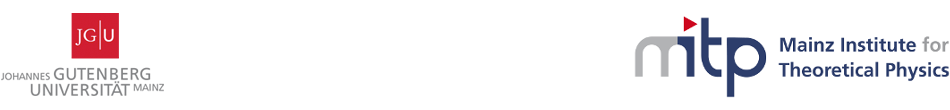Geometry, Gravity and Supersymmetry

24-28 April 2017
Mainz Institute for Theoretical Physics, Johannes Gutenberg University
Europe/Berlin timezone

Tamed symplectic vector bundles for four-dimensional Supergravity

27 Apr 2017, 16:30
30m
02.430 (Mainz Institute for Theoretical Physics, Johannes Gutenberg University)

02.430

Mainz Institute for Theoretical Physics, Johannes Gutenberg University

Staudingerweg 9 / 2nd floor, 55128 Mainz

Speaker

Dr Carlos Shahbazi (Institut für Theoretische Physik, Leibniz Universität Hannover)

Description

I will introduce a system of partial differential equations on a four-manifold M involving a Lorentzian metric coupled to a section of a Lorentzian submersion as well as to a positively-polarized two-form taking values on a tamed flat symplectic vector bundle. I will give a set of sufficient conditions for this system to reduce, when restricted to a contractible open set of M, to the standard local formulas used by physicists in the context of four-dimensional ungauged Supergravity, obtaining thus a mathematically rigorous and globally non-trivial formulation of the latter. We call the resulting theory a "Generalized Einstein-Section-Maxwell theory", or GESM for short. GESM provides a non-trivial extension of standard ungauged supergravity, and its supersymmetrization is expected to yield new Supergravity theories that escape the standard local classification of these theories. Furthermore, I will consider the global automorphism group of a generic GESM theory, giving then a precise definition and characterization of the global U-duality group of the theory. I will also discuss Dirac quantization, which is implemented in terms of a "Dirac system", namely a particular type smooth fiber subbundle of full integral lattices of the symplectic vector bundle of the theory. I will show that generic solutions of GESM theories correspond to the supergravity limit of four-dimensional locally geometric String Theory U-folds, providing some constrains on their global geometry and topology which follow from the global structure of GESM. Finally, I will comment on various open mathematical problems regarding the GESM system of partial differential equations and its moduli space of solutions on a globally hyperbolic Lorentzian four-manifolds. This is based on recent and ongoing work with Calin Lazaroiu.

Summary

I will introduce a system of partial differential equations on a four-manifold M involving a Lorentzian metric coupled to a section of a Lorentzian submersion as well as to a positively-polarized two-form taking values on a tamed flat symplectic vector bundle. I will give a set of sufficient conditions for this system to reduce, when restricted to a contractible open set of M, to the standard local formulas used by physicists in the context of four-dimensional ungauged Supergravity, obtaining thus a mathematically rigorous and globally non-trivial formulation of the latter. We call the resulting theory a "Generalized Einstein-Section-Maxwell theory", or GESM for short. GESM provides a non-trivial extension of standard ungauged supergravity, and its supersymmetrization is expected to yield new Supergravity theories that escape the standard local classification of these theories. Furthermore, I will consider the global automorphism group of a generic GESM theory, giving then a precise definition and characterization of the global U-duality group of the theory. I will also discuss Dirac quantization, which is implemented in terms of a "Dirac system", namely a particular type smooth fiber subbundle of full integral lattices of the symplectic vector bundle of the theory. I will show that generic solutions of GESM theories correspond to the supergravity limit of four-dimensional locally geometric String Theory U-folds, providing some constrains on their global geometry and topology which follow from the global structure of GESM. Finally, I will comment on various open mathematical problems regarding the GESM system of partial differential equations and its moduli space of solutions on a globally hyperbolic Lorentzian four-manifolds. This is based on recent and ongoing work with Calin Lazaroiu.

Primary authors

Dr Calin Lazaroiu (IBS Korea) Dr Carlos Shahbazi (Institut für Theoretische Physik, Leibniz Universität Hannover)

 Slides